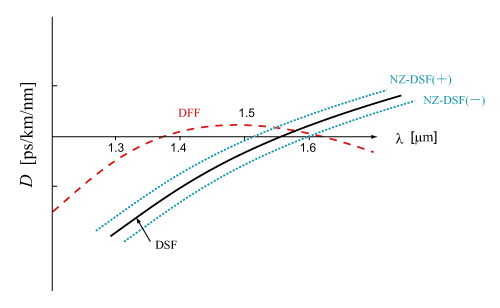By utilizing the property that a waveguide dispersion depends on the parameters such as the core radius and the refractive index difference of core/clad, Δ, it is possible to control the zero dispersion wavelength,λD, and the dispersion slope. Fig.2.17 shows a dispersion characteristics for some of the controlled fibers: dispersion shifted fiber (DSF), nonzero dispersion shifted fiber (NZ-DSF), and dispersion flatted fiber (DFF). All of them is SMF.Figure 2.17: Full dispersion characteristics for dispersion shifted fiber (DSF), nonzero dispersion shifted fiber (NZ-DSF), and dispersion flatted fiber (DFF)

### (1) Dispersion shifted fiber

For the zero dispersion wavelength, λD, to be shifted to ca. 1.55 μm (a wavelength range for optical communication), the optical fiber loss is the minimum. This type of fiber is called as a Dispersion shifted fiber (DSF). DSF is appropriate for uses in long-distance transmission.

### (2) Nonzero dispersion shifted fiber

In a nonzero dispersion shifted fiber (NZ-DSF), the zero dispersion wavelength is slightly shifted from 1550 nm band (usage wavelength range). Then, nonlinear optical phenomena such as four-wave mixing, self-phase modulation, and cross-phase modulation at 1550 nm band is avoided.Therefore, NZ-DSF is useful for wavelength division multiplexing (WDM) using Erbium doped optical fiber amplification (EDFA) and for ultrafast long-distance transmission. In order to avoid a distortion of signal shape yielded by the wavelength dispersion, the value of wavelength dispersion is set to a relatively smaller value compared with a typical fiber.

### (3) Dispersion flatted fiber

Dispersion flatted fiber (DFF) exhibits the zero dispersion at 1.4 μm and 1.6 μm. In DDF, a complex profile of refractive index distribution controls the waveguide dispersion. DDF with a relatively small absolute value of dispersion is appropriate for transmission of ultrashort pulsed light, and can perform a wavelength conversion in the wide wavelength range including C-band range. With using higher nonlinearlity DFF, supercontinuum light with a super wideband spectrum is generated.

### (4) Reverse dispersion fiber

For reverse dispersion fiber (RDF), the absolute values of wavelength dispersion and the dispersion slope are close to those of SMF, but the sign is opposite. Therefore, by combining RDF and SMF with the same fiber length, the wavelength dispersion and the dispersion slope are simultaneously compensated in the whole transmission path, and the zero dispersion is realized in the wide wavelength range (local wavelength dispersion is not zero). Thus, an optical fiber has both positive and negative areas of the wavelength dispersion in the direction of light propagation, and the integrated wavelength dispersion is decreased in the whole transmission path, although the local wavelength dispersion is not zero. This type of optical fiber is called as dispersion management fiber (DMF). DMF is commercially available. Since RDF has a higher nonlinearity than SMF, RDF is arranged at the output side of transmission path in DMF.